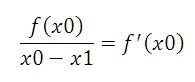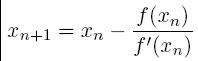# Newton Raphson Method & It's MATLAB Program

Introduction to Iterative methods: There are number of iterative methods like  Jacobi method, Gauss–Seidel method that has been tried and used successfully in various problem situations. All these methods typically generate a sequence of estimates of the solution which is expected to converge to the true solution. Newton-Raphson method is also one of the iterative methods which are used to find the roots of given expression.

### Newton-Raphson Method with MATLAB code:

If point x0 is close to the root a, then a tangent line to the graph of f(x) at x0 is a good approximation the f(x) near a. So the root of the tangent line, where the line cuts the X-axis; x1 is the better approximation to a than x0 is.

Slope of the tangent =ThereforeRepeating process, we obtain a better approximation,Continue in this way. If xn is the current estimate, then the next estimate xn+1 is given byif x0 is sufficiently close to a, xn?a as n?8.

## Limitations of Newton-Raphson Method

1. If initial guess is too far away from the required root, the process may converge to some other root.

2. Division by zero may occur if f’(xi) is zero or very close to zero.

3. A particular value in the iteration sequence may repeat, resulting in an infinite loop.

## Newton-Raphson MATLAB program:

`% Newton Raphson Method clear all close all clc % Change here for different functions f=@(x) cos(x)-3*x+1 %this is the derivative of the above function df=@(x) -sin(x)-3 % Change lower limit 'a' and upper limit 'b' a=0; b=1; x=a; for i=1:1:100 x1=x-(f(x)/df(x)); x=x1; end sol=x; fprintf('Approximate Root is %.15f',sol) a=0;b=1; x=a; er(5)=0; for i=1:1:5 x1=x-(f(x)/df(x)); x=x1; er(i)=x1-sol; end plot(er) xlabel('Number of iterations') ylabel('Error') title('Error Vs. Number of iterations')`

f =
@(x)cos(x)-3*x+1
df =
@(x)-sin(x)-3
Approximate Root is 0.6071016481031231

### You may also like

1.Where value of b=1 is used in this program ; pl let me know; if it is not used anywhere :
what is the necessity;

2.hi my function is f=x-0.2*sind(x)-0.5 but it doesnt work and tells' Undefined function or variable 'x'.'the completed is clear all
close all
clc
% Change here for different functions
f=x-0.2*sind(x)-0.5;
%this is the derivative of the above function
df=1-0.2*cosd(x);
% Change lower limit 'a' and upper limit 'b'
a=0.5; b=1;
x=a;
for i=1:1:100
x1=x-(f(x)/df(x));
x=x1;
end
sol=x;
fprintf('Approximate Root is %.15f',sol)
a=0;b=1;
x=a;
er(5)=0;
for i=1:1:5
x1=x-(f(x)/df(x));
x=x1;
er(i)=x1-sol;
end
plot(er)
xlabel('Number of iterations')
ylabel('Error')
title('Error Vs. Number of iterations') .
3.4.